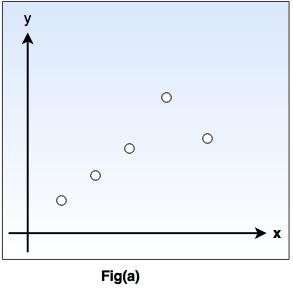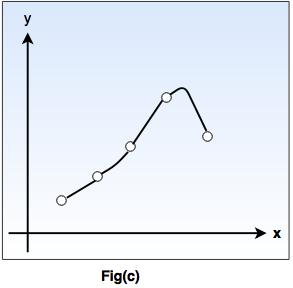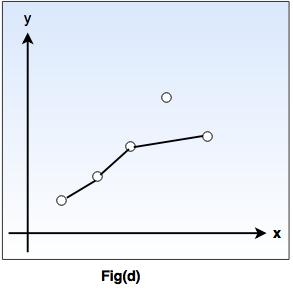# Learning and Expert System in AI

## What is learning?

• According to Herbert Simon, learning denotes changes in a system that enable a system to do the same task more efficiently the next time.
• Arthur Samuel stated that, "Machine learning is the subfield of computer science, that gives computers the ability to learn without being explicitly programmed ".
• In 1997, Mitchell proposed that, " A computer program is said to learn from experience 'E' with respect to some class of tasks 'T' and performance measure 'P', if its performance at tasks in 'T', as measured by 'P', improves with experience E ".
• The main purpose of machine learning is to study and design the algorithms that can be used to produce the predicates from the given dataset.
• Besides these, the machine learning includes the agents percepts  for acting as well as to improve their future performance.
The following tasks must be learned by an agent.

• To predict or decide the result state for an action.
• To know the values for each state(understand which state has high or low vale).
• To keep record of relevant percepts.

#### Why do we require machine learning?

• Machine learning plays an important role in improving and understanding the efficiency of human learning.
• Machine learning is used to discover a new things not known to many human beings.
Various forms of learnings are explained below:

1. Rote learning

• Rote learning is possible on the basis of memorization.
• This technique mainly focuses on memorization by avoiding the inner complexities. So, it becomes possible for the learner to recall the stored knowledge.
For example: When a learner learns a poem or song by reciting or repeating it, without knowing the actual meaning of the poem or song.
2. Induction learning (Learning by example).

• Induction learning is carried out on the basis of supervised learning.
• In this learning process, a general rule is induced by the system from a set of observed instance.
• However, class definitions can be constructed with the help of a classification method.
For Example:
Consider that 'ƒ' is the target function and example is a pair (x ƒ(x)), where 'x' is input and ƒ(x) is the output function applied to 'x'.
Given problem: Find hypothesis h such as h ≈ ƒ

• So, in the following fig-a,  points (x,y) are given in plane so that y = ƒ(x), and the task is to find a function h(x) that fits the point well.

•• In fig-b, a piecewise-linear 'h' function is given, while the fig-c shows more complicated 'h' function.

•• Both the functions agree with the example points, but differ with the values of 'y' assigned to other x inputs.

•• As shown in fig.(d), we have a function that apparently ignores one of the example points, but fits others with a simple function. The true/ is unknown, so there are many choices for h, but without further knowledge, we have no way to prefer  (b), (c), or (d).

• This type is the easiest and simple way of learning.
• In this type of learning, a programmer writes a program to give some instructions to perform a task to the computer. Once it is learned (i.e. programmed), the system will be able to do new things.
• Also, there can be several sources for taking advice such as humans(experts), internet etc.
• However, this type of learning has a more necessity of inference than rote learning.
• As the stored knowledge in knowledge base gets transformed into an operational form, the reliability of the knowledge source is always taken into consideration.

#### Explanation based learning

• Explanation-based learning (EBL) deals with an idea of single-example learning.
• This type of learning usually requires a substantial number of training instances but there are two difficulties in this:
I. it is difficult to have such a number of training instances
ii. Sometimes, it may help us to learn certain things effectively, specially when we have enough knowledge.
Hence, it is clear that instance-based learning is more data-intensive, data-driven while EBL is more knowledge-intensive, knowledge-driven.
• Initially, an EBL system accepts a training example.
• On the basis of the given goal concept, an operationality criteria and domain theory, it "generalizes" the training example to describe the goal concept and to satisfy the operationality criteria (which are usually a set of rules that describe relationships between objects and actions in a domain).
• Thus, several applications are possible for the knowledge acquisition and engineering aspects.

#### Learning in Problem Solving

• Humans have a tendency to learn by solving various real world problems.
• The forms or representation, or the exact entity, problem solving principle is based on reinforcement learning.
• Therefore, repeating certain action results in desirable outcome while the action is avoided if it results into undesirable outcomes.
• As the outcomes have to be evaluated, this type of learning also involves the definition of a utility function. This function shows how much is a particular outcome worth?
• There are several research issues which include the identification of the learning rate, time and algorithm complexity, convergence, representation (frame and qualification problems), handling of uncertainty (ramification problem), adaptivity and "unlearning" etc.
• In reinforcement learning, the system (and thus the developer) know the desirable outcomes but does not know which actions result into desirable outcomes.
• In such a problem or domain, the effects of performing the actions are usually compounded with side-effects. Thus, it becomes impossible to specify the actions to be performed in accordance to the given parameters.
• Q-Learning is the most widely used reinforcement learning algorithm.
• The main part of an algorithm is a simple value iteration update. For each state 'S', from the state set S, and for each action, a, from the action set 'A', it is possible to calculate an update to its expected reduction reward value, with the following expression:

Q(st, at) ←  Q(st, at) + αt (st, at) [rt + γmaxaQ (st+1, a) - Q(st, at)]
• where rt is a real reward at time t, αt(s,a) are the learning rates such that 0 ≤ αt(s,a) ≤ 1, and γ is the discount factor such that 0 ≤ γ < 1.